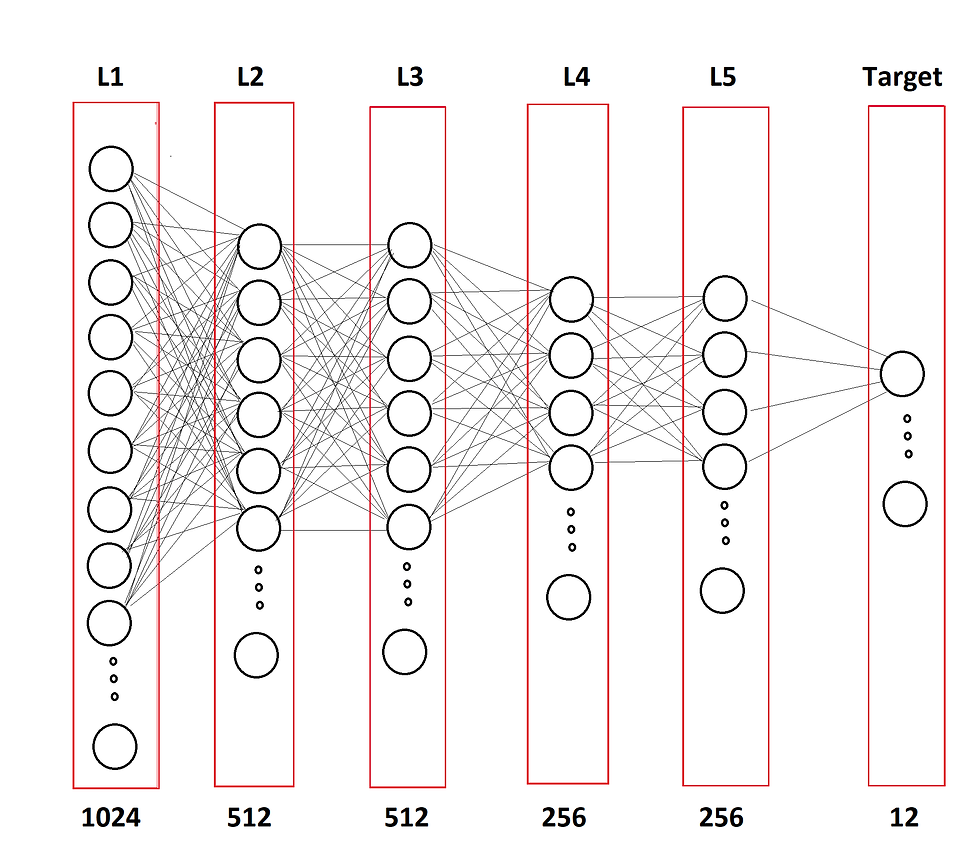Search
• mardania

# CPU vs. GPU : Computation Time Test on Tabular Well Log Data

Machine learning (ML) is growing fast, thanks to computer hardware advancements. The Newer branch of ML, Deep Learning, has been benefited from this emerging technology the most because of parallel computation in GPU’s (Graphical processing units). The purpose of this work is to investigate these question:

• Is Deep Leaning in GPU faster than CPU?

• If Yes, how much?

• Does data size matter?

Dataset

For this test, we used Tabular dataset of Force2010 contest consisting of petrophysical well logs for facies prediction. This is a free data and you may access here. After cleaning and exploratory data analysis, we came up with a table with almost 1,500,000 rows and 28 columns. We ran the main test on this data then for analysis on smaller data, we selected randomly, almost 100,000 row and 14 columns. We kept epoch fixed 30 for whole process as all tests converged before that number.

Hardware and software

All tests has been implemented on Windows 10, using python in Anaconda and Tensorflow 2.4 environment which uses CUDA Toolkit 11 to perform parallel calculation on GPU. The processing units are more specifically an Intel Core i7-10700 (8 cores) @ 2.90-4.8GHz, RAM 32 GB, and Nvidia RTX 3060 Ti GPU.

To memory refresh:

• CPU: The Central Processing Unit is the primary processor for general computation

• GPU: The Graphical Processing Unit consist of several components as the CPU to parallelize the computation.

• CUDA: The Compute Unified Device Architecture is a parallel computing programming platform

Here we do not intend to explain the fundamentals of deep learning. In Deep leaning, matrix (tensor in other language) multiplication is a computationally heavy task that can limit structure and size of networks for learning from the data. This operation can be done in two forms: consecutively (like in CPU) and in parallel (like in GPU). Although GPU’s processing units are weaker than CPU’s with hundreds to thousands Megahertz Clock performance, but several units operation simultaneously in parallel can overcome to CPU’s stronger units. This is achievable in algorithms that can be done in parallel (like deep learning) not consecutive machine learning (like many ML's such as SVM).

Hyper-parameters

The model parameters can be adjusted by the user are called hyper-parameters. For the test of these networks, we have selected batch size and number of dense layers to see how these factors can affect computation speed on both units.

Test1: In this test, it is aimed to examine to see the effect of batch size for training time in both CPU and GPU. A network was constructed in Tensorflow with the structure like the picture below: 5 dense fully connected layers with 1024, 512, 512, 256, and 256 node in each, respectively.Then it was run with various batch sizes ranging from 100 to 100000 batch sizes. The result is plotted below. 'X' axis is the batch size and 'y' axis is computation times in seconds. It is clear that GPU performed faster than CPU. As batch sizes grow, computation time decreases (normally what we expect because larger data size pass to minimization process). Here we do not consider the effect of small and large batch size selection on model generalization. For smaller batches, GPU is 2 times faster than CPU while this can exceed up to 20 times for larger batch sizes. This is because CPU works almost on 100% load capacity in all batch sizes while GPU works in full load in larger batch sizes.For smaller dataset (figure below), for small batch sizes, we see that GPU is less than 2 times faster than CPU while for larger batches it is almost 5 times faster (it is considerably lower than large dataset).Test2: In this test, batch size is set to be 1000 and dense layers varies between 1 to 5. The nodes in each layer is similar to previous test (1024, 512, 512, 256, and 256). As we see in the plot below, GPU is almost 8 times faster than CPU for dense layers of five. For single layer, GPU is just 2 times faster than CPU. It is predictable that, if the nodes quantity reduced for single layer network, GPU and CPU performance will meet each other.For smaller dataset (figure below), this difference diminishes to the half (4 times for 5-layer network and similar for the single layer, respectively).Conclusion

CPU and GPU both can be used for computational purposes in machine learning. In this work, we ran tests to see GPU’s performance on tabular data. Training neural networks on GPU can be considerably faster than CPU if we:

1- deal with large datasets

2- have larger batch sizes for training

3- and complex networks (more hidden dense layers).

In this condition, GPU can be computationally faster (more than 10 times) than CPU. For smaller datasets, batch sizes, and simple networks, GPU is slightly faster than CPU.